预测（评价）模型——层次分析法*

8 篇文章 5 订阅

（最简单的应用是boy们评价美女，girl们评价帅哥哦~）

AHP主要根据问题的性质和要达到的总目标，将问题划分为不同组成因素（简单点说就是小学学的课文，把大问题划分为小问题，然后逐一解决，意思差不多哈~）。并按照因素间的相互关联影响以及隶属关系将因素按不同层次聚集组合，形成一个多层次的分析结构模型，从而最终使问题归结为最低层(供决策的方案、措施等)相对于最高层(总目标)的相对重要权值的确定或相对优劣次序的排定。

1.建立层次结构模型

2.构造判断(成对比较)矩阵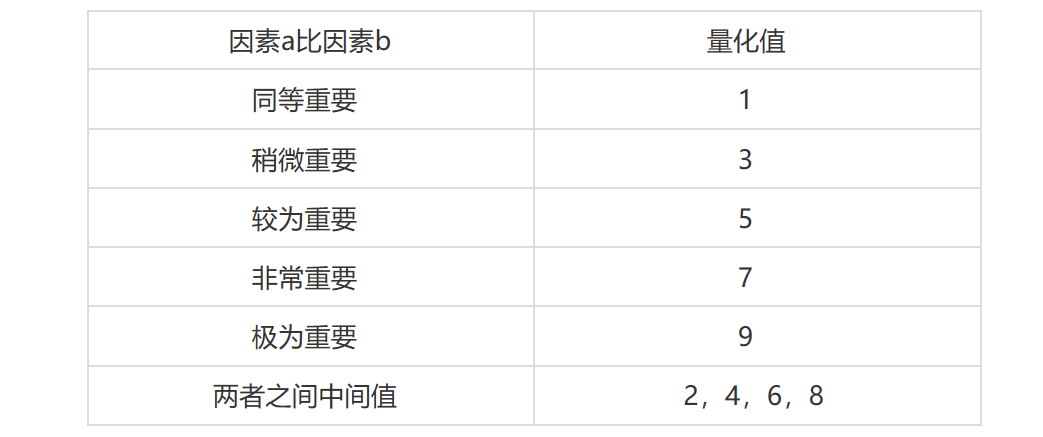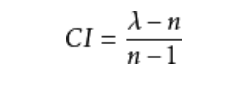CI=0，有完全的一致性；CI 接近于0，有满意的一致性；CI 越大，不一致越严重。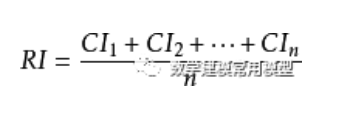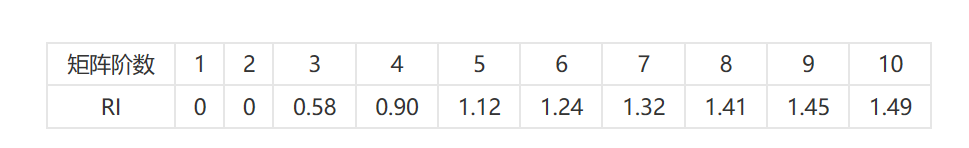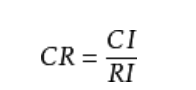4.层次总排序及其一致性检验

—— —— —— —— —— —— —— —— —— —— —— —— —— —介绍就这么多我们拿具体的例题来讲具体计算步骤以最简单的例子，假设我们要买一根钢笔首先我们明确 比例标度表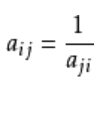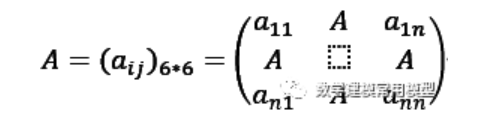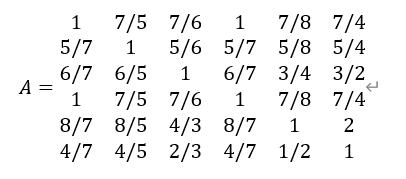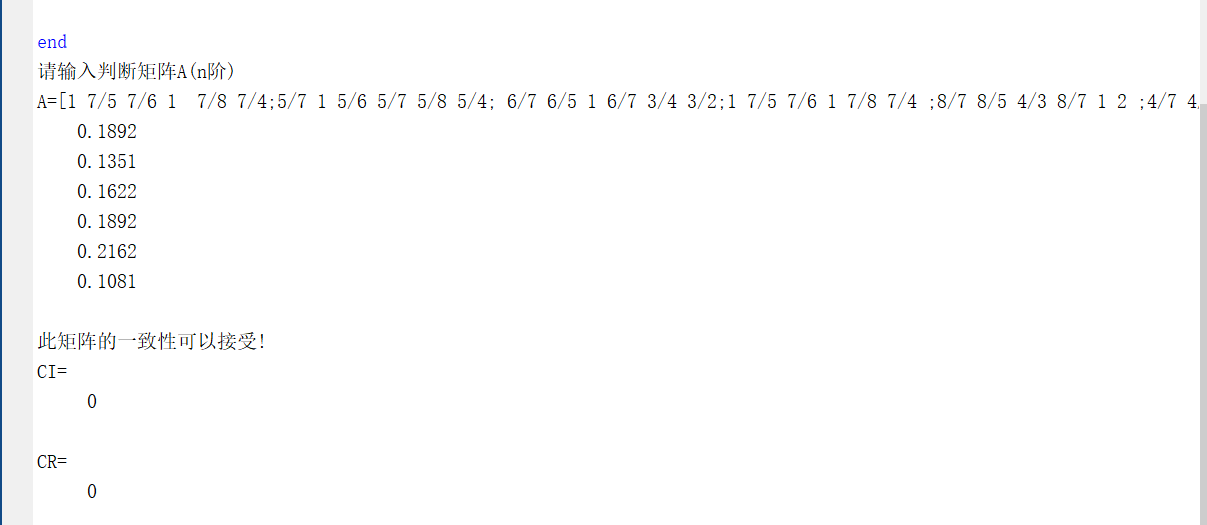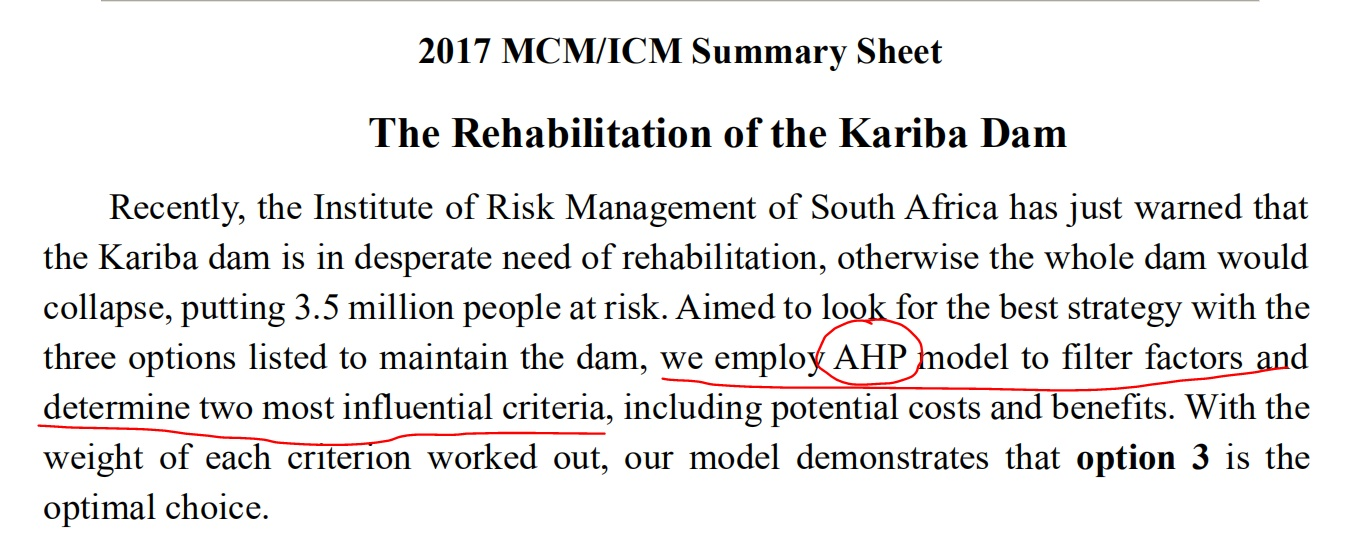disp('请输入判断矩阵A（n阶）');
A=input('A=');
[n,n]=size(A);
x=ones(n,100);
y=ones(n,100);
m=zeros(1,100);
m(1)=max(x(:,1));
y(:,1)=x(:,1);
x(:,2)=A*y(:,1);
m(2)=max(x(:,2));
y(:,2)=x(:,2)/m(2)
;p=0.0001;i=2;k=abs(m(2)-m(1));
while  k>p
i=i+1;
x(:,i)=A*y(:,i-1);
m(i)=max(x(:,i));  y(:,i)=x(:,i)/m(i);
k=abs(m(i)-m(i-1));
end
a=sum(y(:,i));
w=y(:,i)/a;
t=m(i);disp(w);
%以下是一致性检验
CI=(t-n)/(n-1);RI=[0 0 0.52 0.89 1.12 1.26 1.36 1.41 1.46 1.49 1.52 1.54 1.56 1.58 1.59];
CR=CI/RI(n);if CR<0.10
disp('此矩阵的一致性可以接受!');
disp('CI=');disp(CI);
disp('CR=');disp(CR);
end
————————————————

01-292460

02-111万+
10-17
06-26
04-144835
11-12370
01-271477
08-313万+
07-23268
01-10
12-111万+
08-267076
01-189573
09-22220
10-102690
07-222377¥2 ¥4 ¥6 ¥10 ¥20余额支付 (余额：-- )扫码支付获取中扫码支付点击重新获取扫码支付1.余额是钱包充值的虚拟货币，按照1:1的比例进行支付金额的抵扣。
2.余额无法直接购买下载，可以购买VIP、C币套餐、付费专栏及课程。余额充值